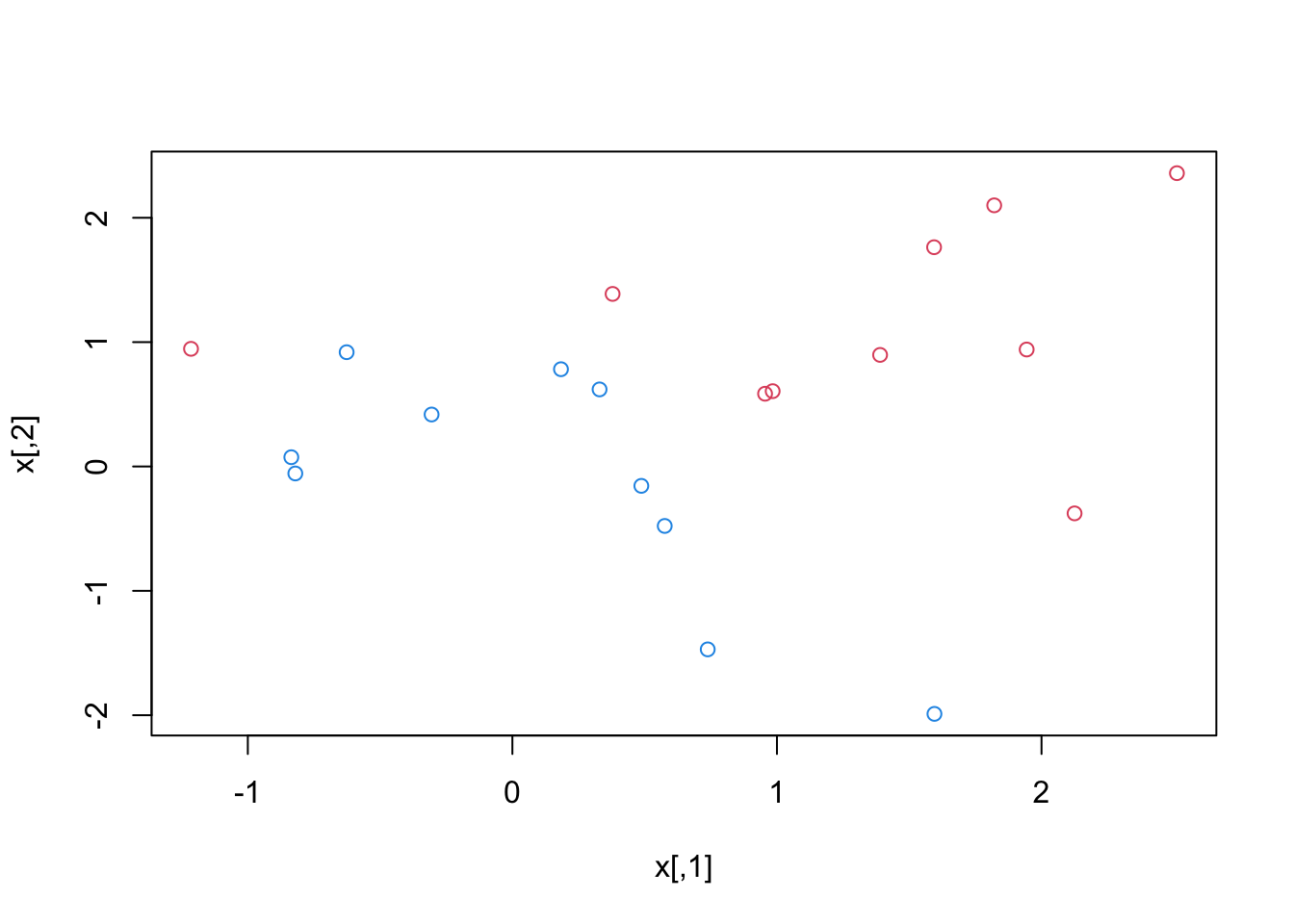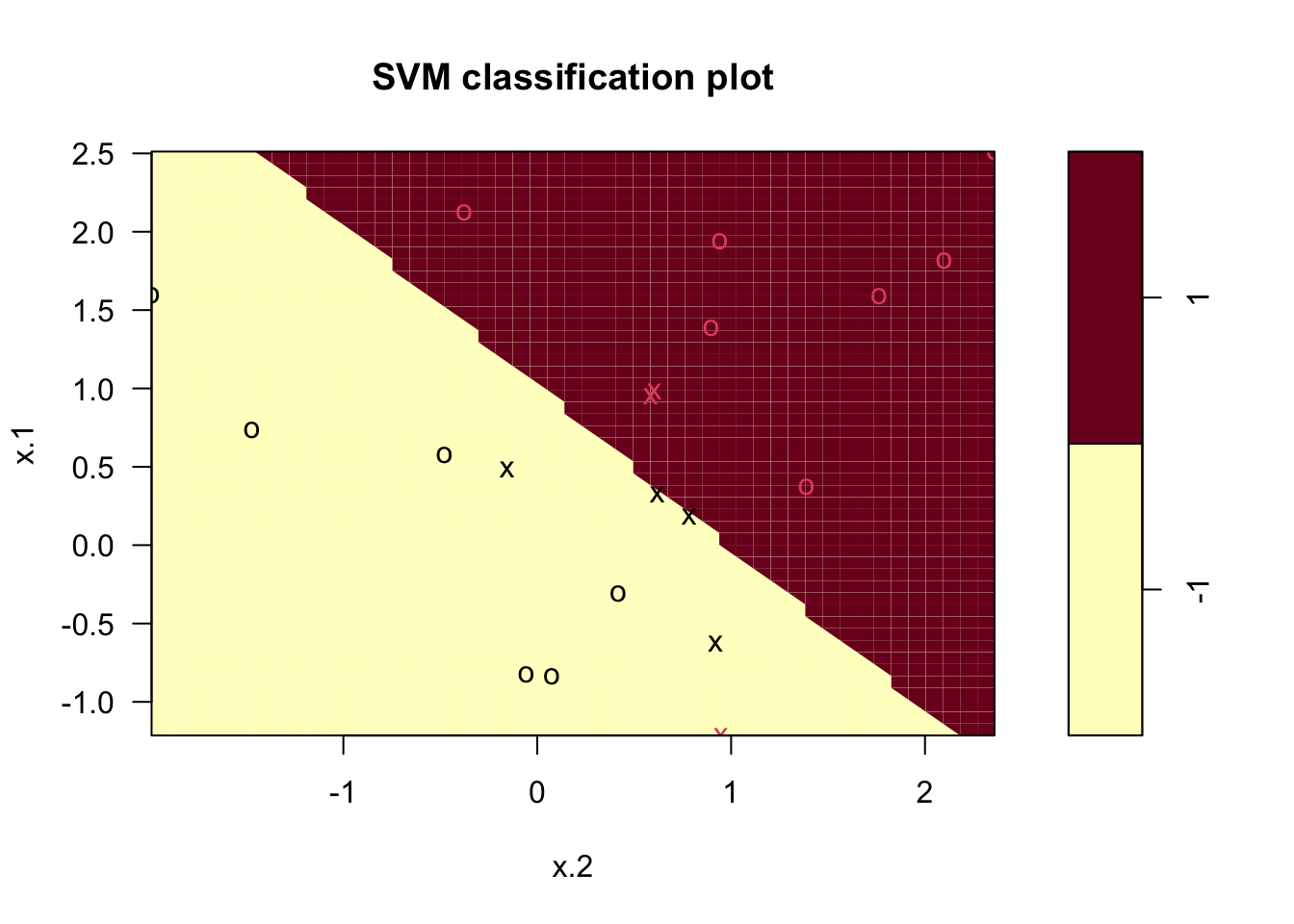ISLR Home

p359

# Support Vector Classifier

We begin by generating the observations, which belong to two classes, and checking whether the classes are linearly separable.

``````set.seed(1)
x=matrix(rnorm(20*2), ncol=2)
y=c(rep(-1,10), rep(1,10))
x[y==1,]=x[y==1,] + 1
plot(x, col=(3-y))``````They are not.

We fit the support vector classifier. Note that in order for the svm() function to perform classification (as opposed to SVM-based regression), we must encode the response as a factor variable. We now create a data frame with the response coded as a factor.

The argument scale=FALSE tells the svm() function not to scale each feature to have mean zero or standard deviation one;

``````dat=data.frame(x=x, y=as.factor(y))
library(e1071)
svmfit=svm(y~., data=dat, kernel="linear", cost=10, scale=FALSE)``````

Plot the support vector classifier

Note that here the second feature is plotted on the x-axis and the first feature is plotted on the y-axis, in contrast to the behavior of the usual plot() function in R.

``plot(svmfit, dat)``7 support vectors

``svmfit\$index``
``##   1  2  5  7 14 16 17``
``summary(svmfit)``
``````##
## Call:
## svm(formula = y ~ ., data = dat, kernel = "linear", cost = 10, scale = FALSE)
##
##
## Parameters:
##    SVM-Type:  C-classification
##  SVM-Kernel:  linear
##        cost:  10
##
## Number of Support Vectors:  7
##
##  ( 4 3 )
##
##
## Number of Classes:  2
##
## Levels:
##  -1 1``````

There were seven support vectors, four in one class and three in the other.

Observations that lie directly on the margin, or on the wrong side of the margin for their class, are known as support vectors